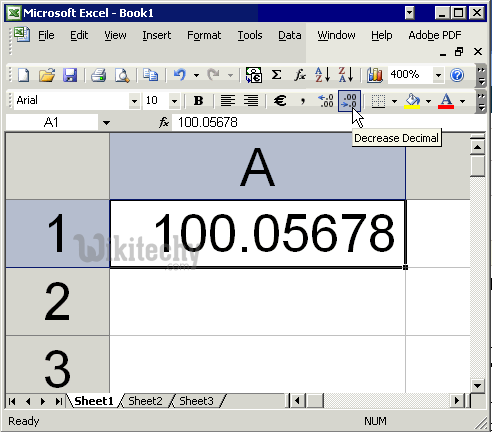# pig tutorial - apache pig tutorial - Apache Pig ROUND() - pig latin - apache pig - pig hadoop

## What is ROUND() function in Apache Pig ?

• The ROUND() function returns a number rounded to a given number of digits.
• The ROUND() function can round to the right or left of the decimal point.
• The ROUND() function is used to get the value of an expression rounded to an integer (if the result type is float) or rounded to a long (if the result type is double).Learn Apache Pig - Apache Pig tutorial - Apache Pig round - Apache Pig examples - Apache Pig programs

## Example

• Ensure that we have a file named wikitechy_math.txt in the HDFS directory /pig_data/.
• This file contains integer and floating point values as given below.

wikitechy_math.txt

• You have loaded this file into Pig with a relation named math_data as given below.
• Now you can calculate round values of the contents of the wikitechy_math.txt file using ROUND() function as given below.

## Verification

• Verify the contents of the relation using the Dump operator as given below.

## Output

• · The above statement stores the result in the relation named round_data.